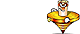Unit Conversion Software Web Widgets Loan Calculator Currency Rates Country Flags Unit Converter
 Faq HelpNewton Meter ConversionContact

Newton Meter, unit of measure

To perform conversions between newton meter and other Torque units please try our Torque Unit Converter

Convert newton meter to:
newton centimeter, newton millimeter, kilonewton meter, dyne meter, dyne centimeter, dyne millimeter, kilogram-force meter, kilogram-force centimeter, kilogram-force millimeter, gram-force meter, gram-force centimeter, gram-force millimeter, ounce-force foot, ounce-force inch, pound-force foot, pound-force inchDownload Torque Unit Converter
our powerful software utility that helps you make easy conversion between more than 2,100 various units of measure in more than 70 categories. Discover a universal assistant for all of your unit conversion needs - download the free demo version right away!Copyright © 1998-2009 UnitConversion.org Privacy & Terms | About | Faq | Help | Contact | Link to Us